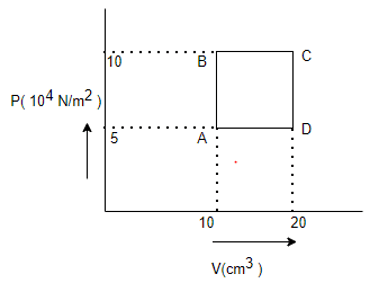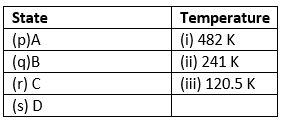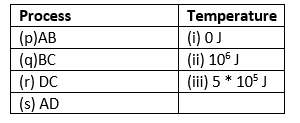# Online Test for Class 11 Physics Thermodynamics

General Instructions

1. Your test contains multiple-choice questions with only one answer type questions. There are a total of 20 questions
2. This is a 30 min test. Please make sure you complete it in stipulated time
3. You can finish this test any time using the 'Submit' button.

1. Which one of the following is incorrect?
2. In a cyclic process, work done by the system is
3. The efficiency of a carnot engine when source temperature is  $T_1$ and sink temperature in $T_2$ will be
4. An ideal gas at 27&deg; C has compressed adiabatically to 8/27 of its initial volume. If the $\gamma=5/3$, then the rise in temperature is
5. The door of a domestic refrigerator is kept open while the switch is on. Then the room will
6. which of the following is not a thermodynamical function?
7. Universal gas constant is
8. Starting with the same initial conditions, an ideal gas expands from volume V1 to V2 in three different ways: The work done by the gas is W1, if the process is purely isothermal; W2, if the process is purely isobaric and W3, if the process is purely adiabatic, then
9. The efficiency of Carnot engine is .6. It rejects 20J of heat to Sink. The work done by the engine
10. Calculate the work done if temperature is changed  from 0&deg; C to 200&deg;C at one temperature (R=2 cal/K)
11. In the following questions, a statement of assertion is followed by a statement of reason. You are required to choose the correct one out of the given four responses and mark it as
(a) If both assertion and reason are true and reason is the correct explanation of the assertion.
(b) If both assertion and reason are true but reason is not correct explanation of the assertion.
(c) If assertion is true, but reason is false.
(d) If both assertion and reason are false.
(e) If reason is true but assertion is false.Assertion: Reversible systems are difficult to fine in real world.
Reason: Most processes are dissipative in nature.
12. In the following questions, a statement of assertion is followed by a statement of reason. You are required to choose the correct one out of the given four responses and mark it as
(a) If both assertion and reason are true and reason is the correct explanation of the assertion.
(b) If both assertion and reason are true but reason is not correct explanation of the assertion.
(c) If assertion is true, but reason is false.
(d) If both assertion and reason are false.
(e) If reason is true but assertion is false.Assertion: In an adiabatic process change in internal energy of a gas in equal to work done on or by the gas in the process
Reason: Temperature of gas remains constant in an adiabatic process.
13. In the following questions, a statement of assertion is followed by a statement of reason. You are required to choose the correct one out of the given four responses and mark it as
(a) If both assertion and reason are true and reason is the correct explanation of the assertion.
(b) If both assertion and reason are true but reason is not correct explanation of the assertion.
(c) If assertion is true, but reason is false.
(d) If both assertion and reason are false.
(e) If reason is true but assertion is false.Assertion: The melting of solid causes an increase in the internal energy
Reason: Latent heat is the heat required to melt a unit mass of the solid
14. In the following questions, a statement of assertion is followed by a statement of reason. You are required to choose the correct one out of the given four responses and mark it as
(a) If both assertion and reason are true and reason is the correct explanation of the assertion.
(b) If both assertion and reason are true but reason is not correct explanation of the assertion.
(c) If assertion is true, but reason is false.
(d) If both assertion and reason are false.
(e) If reason is true but assertion is false.Assertion: For an isothermal process in an ideal gas, the heat absorbed by the gas is entirely used in the work done by the gas.
Reason: During a process taking place in a system, the temperature remains constant then the process is isothermal.
15. A sample of 2kg of monoatomic Helium (assume ideal) is taken through the process ABC and another sample of 2 kg of the same gas is taken through the process ADC. Given, relative molecular mass of Helium =4.

Given Molecular mass of Helium= 4 and R=8.3 J/mol KMatch the column16. Match the column Work done17. Heat Involved in the Process ABC
18. Heat Involved in the Process ADC
19. A perfect gas is contained in a cylinder kept in vacuum. If the cylinder suddenly bursts, then the temperature of the gas
20. For Hydrogen gas $C_P -C_V=a$  and for oxygen gas $C_P-C_V=b$, so the relation between a and b is given by

Subscribe
Notify ofThis site uses Akismet to reduce spam. Learn how your comment data is processed.